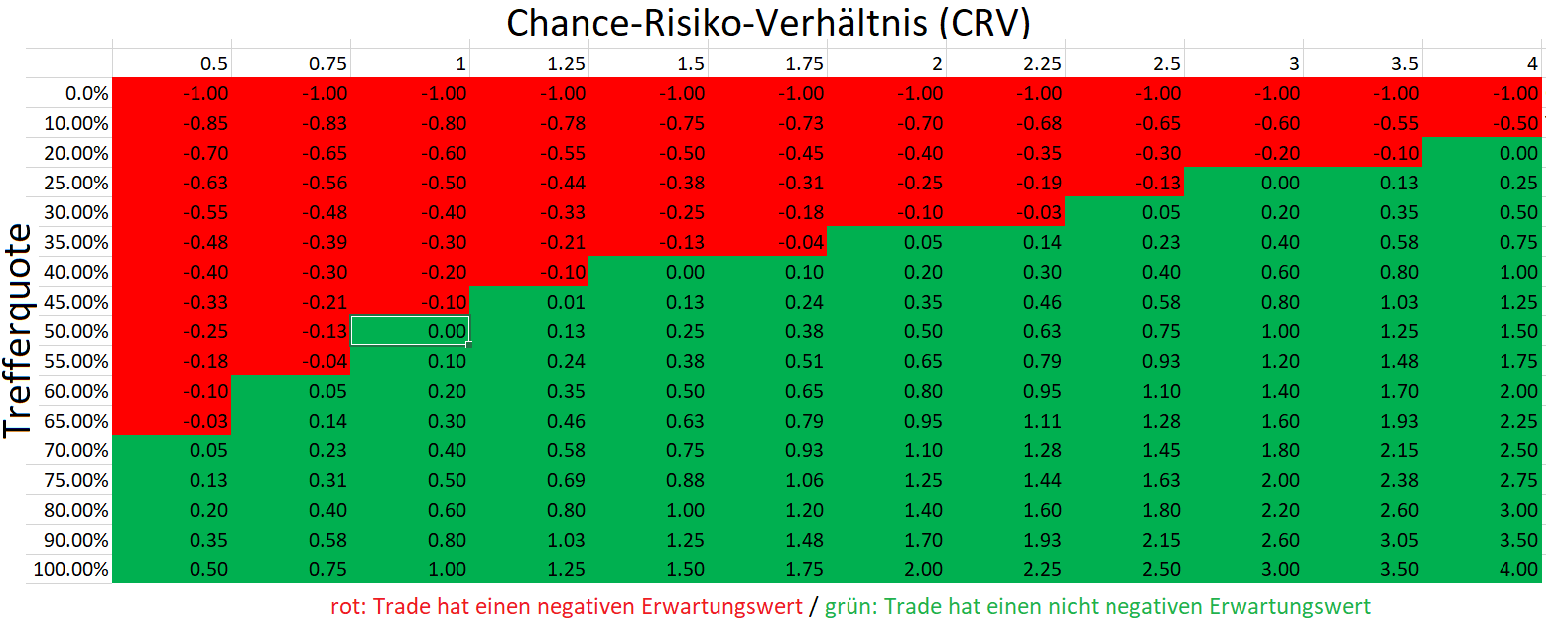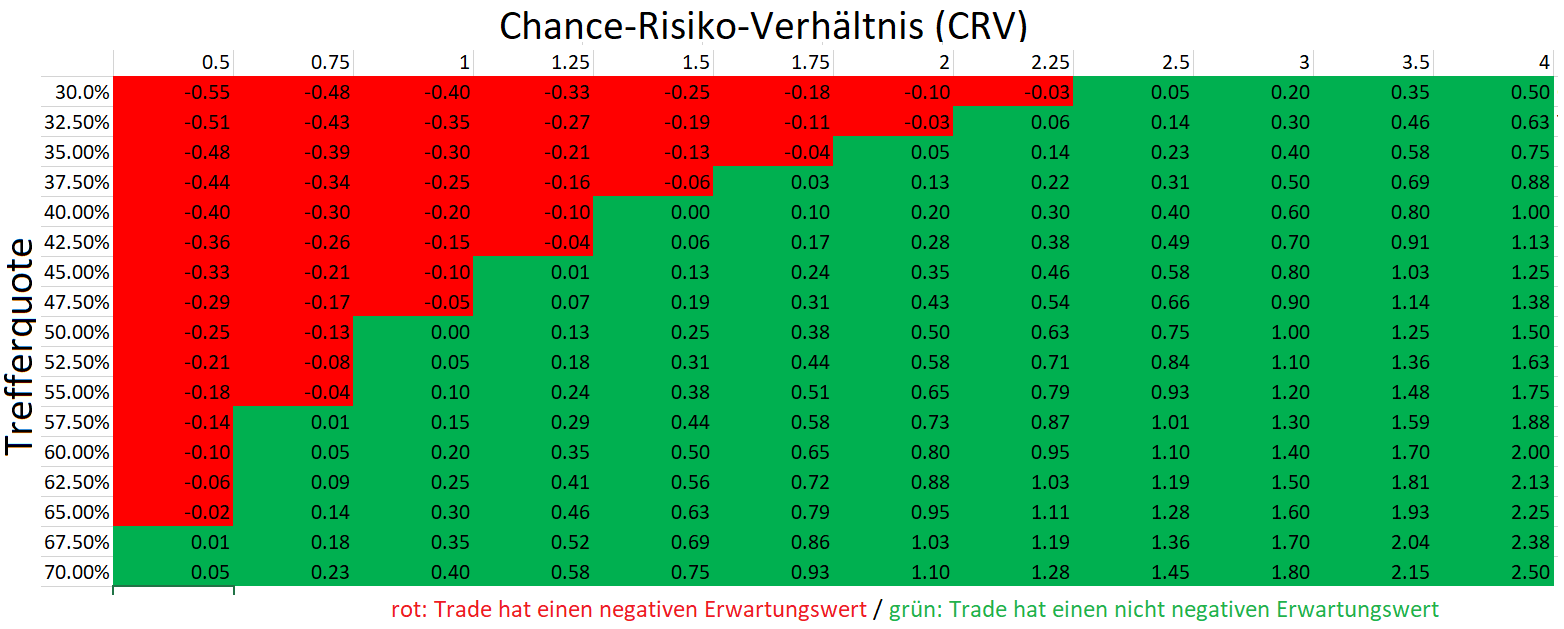# CRV and hit rate: What every trader needs to know

If you want to make money with short-term trading on the stock exchange, you should take a closer look at the risk-reward ratio (CRV) and the hit rate as well as the relationship between the two variables.

To Risk-Reward Ratio (CRV) indicates how large the possible gain is in relation to the possible loss. For example, a CRV of 2. means that the profit on success is twice as large as the loss on loss. With a CRV of 1, the potential gain and the potential loss are exactly the same. With a CRV of 0.5, the potential gain is only half the potential loss. A specific CRV cannot be specified in advance for all trades. This is especially true if it is not clear from the outset at what level the trader will leave in case of loss (stop loss) or in case of profit (take profit). In such cases, however, one can calculate an average CRV over many similar trades. An average CRV of 2 means that the gains on average are double the losses.

The second elementary goal of a trading setup or strategy is hitrate. The hit rate is the probability of winning. A hit rate of 50% means that there is a gain half the time. A hit rate of 25% means that only a quarter of all trades end up with profits. Contrary to many beginners’ beliefs, a trading strategy with a hit rate of less than 50% can be profitable. Although most trades then result in losses, the profits from winning trades can be so large in proportion that they can more than compensate for the many small losses.

CRV and hit rate are strongly negatively correlated with each other. This means that a high CRV is usually associated with a low hit rate and a high hit rate with a low CRV.

trend-following approaches often has a low hit rate but a high CRV: Although many trades end up with losses, a few trades make such large profits that they ideally more than compensate for the many small losses.

Too anticyclical rebound strategies the opposite is true: These strategies often have a relatively high hit rate but a poor CRV. Many trades end up with profits, but the profits per. trading is small compared to the (rather rare) losses.

Special care is required with strongly asymmetric strategies with a very high hit rate and a very low CRV. These strategies yield small profits on almost every trade and only very rarely losses, which can then be huge. Here, to the untrained observer, it looks as if the strategy is a money printing machine. In truth, however, the risk of a total loss always lurks over the trader. Such a strategy was presumably implemented in the strategy in the following diagram (source: Wikifolio). Many small and regular gains are characterized by few but large losses that occur very rarely.

The following two tables show the so-called expected value for profit for trades with different hit rates and CRVs. It is assumed that there is only one winning and one losing scenario, which is characterized by the hit rate and the probability.The expected value for profit given in the tables indicates how much a trader would earn or lose per. invested euros if he performed many similar trades with the corresponding hit rate and the corresponding CRV.If a trader performs many trades with a hit rate of 50% and a CRV of 1 (potential profit equals potential loss), the bottom line is that he neither wins nor loses anything (expected value for profit is 0). However, if the trader has discovered a strategy where he is right 52.5% of the time and wrong 47.5% of the time, he will earn an additional 10 cents per hour. bet dollars in the long run if CRV is 1.

In reality, there are only a few short-term strategies or setups where the expected value differs very significantly from 0. For example, a trading strategy with a hit rate of 95% and a CRV of 1 is pure fiction, just like a CRV of 4 with a 50% probability is. On the other hand, with a CRV of 1, a hit rate of just over 50% is enough to generate long-term profit.

Tip: Test now Instructions PROmax! There you will find lots of trading ideas, sample portfolios, a direct exchange with our stock exchange experts in a special stream and exciting tools such as the formula editor or the stock screener. Goodmode PLUS is also included. Test the new PROmax now for 14 days for free!